# JP4357967B2 - Control device for synchronous reluctance motor - Google Patents

## Info

Publication number
JP4357967B2
JP4357967B2 JP2003579355A JP2003579355A JP4357967B2 JP 4357967 B2 JP4357967 B2 JP 4357967B2 JP 2003579355 A JP2003579355 A JP 2003579355A JP 2003579355 A JP2003579355 A JP 2003579355A JP 4357967 B2 JP4357967 B2 JP 4357967B2
Authority
JP
Japan
Prior art keywords
value
synchronous reluctance
command value
reluctance motor
means
Prior art date
Legal status (The legal status is an assumption and is not a legal conclusion. Google has not performed a legal analysis and makes no representation as to the accuracy of the status listed.)
Expired - Fee Related
Application number
JP2003579355A
Other languages
Japanese (ja)
Other versions
JPWO2003081765A1 (en
Inventor

Original Assignee
パナソニック株式会社
Priority date (The priority date is an assumption and is not a legal conclusion. Google has not performed a legal analysis and makes no representation as to the accuracy of the date listed.)
Filing date
Publication date
Priority to JP2002081171 priority Critical
Priority to JP2002081171 priority
Application filed by パナソニック株式会社 filed Critical パナソニック株式会社
Priority to PCT/JP2003/003419 priority patent/WO2003081765A1/en
Publication of JPWO2003081765A1 publication Critical patent/JPWO2003081765A1/en
Application granted granted Critical
Publication of JP4357967B2 publication Critical patent/JP4357967B2/en
Expired - Fee Related legal-status Critical Current
Anticipated expiration legal-status Critical

• 230000001360 synchronised Effects 0.000 title claims description 166
• 238000006243 chemical reactions Methods 0.000 claims description 6
• 230000003247 decreasing Effects 0.000 claims description 4
• 230000002123 temporal effects Effects 0.000 claims 3
• 230000001939 inductive effects Effects 0.000 claims 1
• 230000001629 suppression Effects 0.000 description 6

## Images

•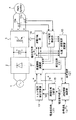•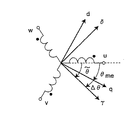•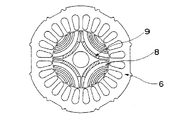•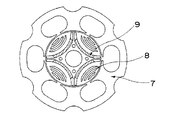••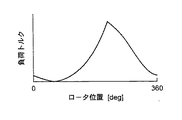••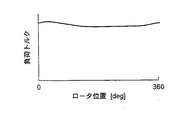•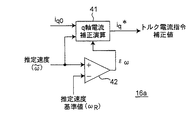•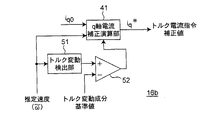•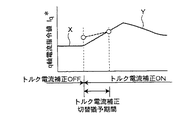•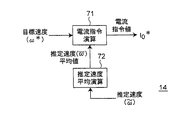•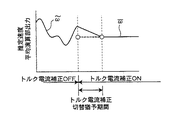••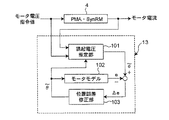••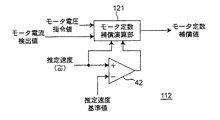•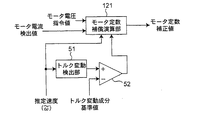•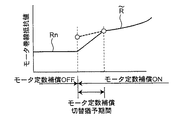•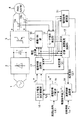•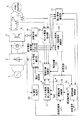••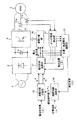•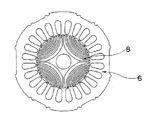## Classifications

• HELECTRICITY
• H02GENERATION; CONVERSION OR DISTRIBUTION OF ELECTRIC POWER
• H02PCONTROL OR REGULATION OF ELECTRIC MOTORS, ELECTRIC GENERATORS OR DYNAMO-ELECTRIC CONVERTERS; CONTROLLING TRANSFORMERS, REACTORS OR CHOKE COILS
• H02P25/00Arrangements or methods for the control of AC motors characterised by the kind of AC motor or by structural details
• H02P25/02Arrangements or methods for the control of AC motors characterised by the kind of AC motor or by structural details characterised by the kind of motor
• H02P25/08Reluctance motors
• H02P25/092Converters specially adapted for controlling reluctance motors
• HELECTRICITY
• H02GENERATION; CONVERSION OR DISTRIBUTION OF ELECTRIC POWER
• H02PCONTROL OR REGULATION OF ELECTRIC MOTORS, ELECTRIC GENERATORS OR DYNAMO-ELECTRIC CONVERTERS; CONTROLLING TRANSFORMERS, REACTORS OR CHOKE COILS
• H02P25/00Arrangements or methods for the control of AC motors characterised by the kind of AC motor or by structural details
• H02P25/02Arrangements or methods for the control of AC motors characterised by the kind of AC motor or by structural details characterised by the kind of motor
• H02P25/08Reluctance motors
• H02P25/086Commutation
• H02P25/089Sensorless control

## Description


BACKGROUND OF THE INVENTION
The present invention relates to a control device for a synchronous reluctance motor, and more particularly to a control device that estimates and drives an angle of a rotor without using a position sensor.

[Prior art]
Conventional control devices for synchronous reluctance motors obtain rotor angle information using position sensors such as Hall elements, resolvers, or optical encoders. Therefore, the cost has increased by the position sensor, and the size of the synchronous reluctance motor has increased.

Therefore, a control device for a synchronous reluctance motor as shown in FIG. 23 is generally known as a control device for a synchronous reluctance motor that realizes low cost and size reduction by omitting the position sensor.

In FIG. 23, the main circuit includes an AC power source 1, an AC / DC converter 2 that converts AC power into DC power, a DC / AC converter 3 that converts DC power into AC power, and a DC / AC converter 3 It is comprised from the synchronous reluctance motor 5 driven with the alternating current power converted by (3).

On the other hand, the control circuit includes current detectors 11a and 11b and a motor current detection unit 12 for detecting the motor current, a position / speed estimation calculation unit 13 for estimating the position and speed of the synchronous reluctance motor, and an external application. A speed control calculation unit 14 for determining a current command value so that a speed error between a speed command value obtained and a speed estimation value obtained from the position / speed estimation calculation unit 13 is zero; and a current command obtained from the speed control calculation unit 14 The energization phase distribution unit 15 that distributes the value to the torque current component and the field current component, and the voltage command value is determined so that the current error between the torque current command value, the field current command value, and the motor current detection value is zero. The current control calculation unit 17 and an energization distribution unit 18 that distributes an energization signal for each drive element in the synchronous reluctance motor 5 are configured.

FIG. 24 is a cross-sectional view showing a configuration of a general synchronous reluctance motor 5. The synchronous reluctance motor 5 includes a rotor 8 and a stator 6.

In the control device shown in FIG. 23, the position / speed estimation calculation unit 13 obtains the magnetic flux using information on the motor current and the voltage command value. Next, an αβ-axis magnetic flux angle indicating an angle of the magnetic flux with respect to the stationary coordinate is obtained. Then, a dq axis coordinate phase indicating the phase of the magnetic flux with respect to the rotation coordinate is set. Further, the estimated angle is obtained by subtracting the dq-axis coordinate phase from the αβ-axis coordinate angle. And the synchronous reluctance motor 5 is controlled based on this estimated angle.

In addition, two types of angle estimation are performed: low-speed angle estimation and high-speed angle estimation. At the boundary between the low-speed range and the high-speed range, the angle ratio estimated by both methods is gradually changed and combined to generate an estimated angle. To do. In the low speed range, a current pulse is applied and the angle is obtained from the voltage response.

For example, a position sensorless drive type synchronous reluctance motor described in Japanese Patent Application Laid-Open No. 2001-197774 has low-pass filter means for applying a low-pass function to a voltage command value, and the action of the low-pass function is increased when the rotor speed increases. By making it small, the influence of voltage pulses is removed at the boundary between the low speed range and the high speed range, and the angle estimation method is switched stably, and control of a synchronous reluctance motor without time delay in the high speed range is realized. Yes.

[Problems to be solved by the invention]
However, in the above conventional configuration, the control calculation is complicated, and when the voltage control rate of the inverter exceeds 100%, it is difficult to estimate the position and speed when so-called voltage saturation occurs or when there is a sudden load fluctuation. Thus, there has been a problem that motor drive control becomes unstable.

The present invention solves such a conventional problem, and an object of the present invention is to provide a control device for a synchronous reluctance motor in which a control system that is robust against voltage saturation and sudden load fluctuations is constructed.

[Means for Solving the Problems]
The motor control device of the present invention is a device that controls a synchronous reluctance motor that uses at least a change in inductance of a stator winding and a reluctance torque generated in accordance with a motor current.

In the control device, the current detection means detects the motor current flowing in the stator winding of the synchronous reluctance motor.

The position / speed estimation means estimates the induced voltage of the synchronous reluctance motor from the detection value by the current detection means and the voltage command value which is the command value of the voltage applied to the stator winding of the synchronous reluctance motor. Based on the estimated value of the induced voltage, the estimated value of the rotor position and rotational speed of the synchronous reluctance motor is determined.

The speed control means is a current command supplied to the stator winding of the synchronous reluctance motor so that the error between the estimated value of the rotational speed by the position / speed estimation means and the target value of the rotational speed given from the outside is zero. Determine the value.

The distribution means determines the current command value from the speed control means based on a preset current phase angle of the synchronous reluctance motor, the torque current command value that is the torque current component, and the field current command value that is the field current component. To distribute.

The torque current correction means is obtained from the torque current command value from the distribution means and the position / speed estimation means so that the load torque generated by the load element of the synchronous reluctance motor matches the output torque of the synchronous reluctance motor. The torque current command value is corrected based on the estimated value of the rotational speed.

The current control means sets the error between the correction value of the torque current command value from the torque current correction means and the field current command value from the distribution means and the detection value of the motor current obtained from the current detection means to be zero. Generate a voltage command value.

The energization distribution unit distributes the energization signal to each drive element in the synchronous reluctance motor based on the voltage command value.

The control device of the present invention has the above-described configuration, so that the load torque generated by the load element of the synchronous reluctance motor is always matched with the output torque of the synchronous reluctance motor. As a result, a control system that is robust against steep load fluctuations can be realized, and torque fluctuations can be suppressed to achieve low vibration and low noise.

DETAILED DESCRIPTION OF THE INVENTION
Hereinafter, a preferred embodiment of a control device for a synchronous reluctance motor according to the present invention will be described with reference to the accompanying drawings.

(Embodiment 1)
FIG. 1 shows a system configuration diagram of an embodiment of a control device for a synchronous reluctance motor according to the present invention. In the following, an example in which sinusoidal driving that is 180-degree energization is performed without using a position sensor for a permanent magnet-assisted synchronous reluctance motor will be described.

The main circuit is converted by an AC power source 1, an AC / DC converter 2 that converts AC power into DC power, a DC / AC converter 3 that converts DC power into AC power, and a DC / AC converter 3. It is comprised from the permanent magnet auxiliary | assistant type synchronous reluctance motor 4 driven by alternating current power.

The control circuit includes current detectors 11a and 11b and a motor current detection unit 12 for detecting a motor current, a position / speed estimation calculation unit 13 for estimating the position and speed of a permanent magnet auxiliary synchronous reluctance motor, Obtained from the speed control calculation unit 14 and the speed control calculation unit 14 for determining the current command value so that the speed error between the speed command value given from the position and the speed estimation value obtained from the position / speed estimation calculation unit 13 is zero. An energization phase distribution unit 15 that distributes the current command value into a torque current component and a field current component, a torque current correction calculation unit 16 that corrects the torque current command value, a correction value of the torque current command value, and a field current command value A current control calculation unit 17 for determining a voltage command value so that a current error between the motor current detection value and the motor current detection value becomes zero, and a permanent magnet auxiliary synchronous reluctance motor And a power distributor 18 for distributing the conduction signal for each drive element in 4.

FIG. 2 is a diagram showing the definition of coordinate axes in position / velocity estimation. In general, when sinusoidal driving is performed, various amounts of the motor are changed from three phases u, v, and w to two phases of the dq axis as shown in FIG. Perform phase conversion and convert to direct current. In addition, about the conversion method from three phases to two phases, since it is well-known, it abbreviate | omits. In FIG. 2, θmeIs the actual rotor position (phase difference from the u-phase reference q-axis), and θ ~ is the estimated position (phase difference from the u-phase reference γ-axis). Further, the positional error Δθ has the following relationship.
Δθ = θ ~ −θme

FIGS. 3 and 4 are diagrams showing a configuration example of the permanent magnet auxiliary type synchronous reluctance motor 4. The synchronous reluctance motor shown in FIG. 3 includes a distributed winding stator 6 and a rotor 8 having permanent magnets 9. The synchronous reluctance motor shown in FIG. 4 includes a concentrated winding stator 7 and a rotor 8 having permanent magnets 9. Motor efficiency is improved by incorporating a permanent magnet in the rotor. As the permanent magnet 9, it is preferable to use a permanent magnet having only a minimum magnetic amount necessary to cancel the brake torque at the rated load of the synchronous reluctance motor. As a result, it is possible not only to improve the motor efficiency at the rated load, but also to suppress the cost increase due to the permanent magnet to a minimum.

An example of the magnetic flux vector of the permanent magnet auxiliary synchronous reluctance motor 4 is shown in FIG. In FIG. 5, a vector A is a magnetic flux component that generates a positive torque, and a magnetic flux component (vector C) of the auxiliary magnet acts to cancel a magnetic flux component (vector B) that generates a brake torque (negative torque). Note that the generated torque in this case is expressed as shown in Equation (1).

[Expression 1]
In equation (1), np is the number of pole pairs, Ld, LqIs the d-axis and q-axis inductance Λ0 is the number of flux linkages by the permanent magnet, and id and iq are the d-axis and q-axis currents, respectively.

Hereinafter, position sensorless driving of the permanent magnet auxiliary synchronous reluctance motor 4 will be described.

First, the speed control calculation unit 14 receives a rotational speed command value ω given from the outside.*And the rotational speed estimated value ω ~, the current command value I*Ask for.

[Expression 2]
Where Kp1, KI1Is the gain of the PI compensator, and p is the differential operator.

Next, the energization phase distribution unit 15 determines the current command value I*And the current phase angle β set in advance and the current command value I*D-axis current id *And q-axis current iq0To distribute.

[Equation 3]

The torque current correction calculation unit 16 calculates the rotational speed estimated value ω ~ and the q-axis current i.q0Q-axis current command value i by the calculation represented by the following equationq *Is derived.

[Expression 4]
Here, in Equation (4), a discrete time system is used in order to perform an operation by a microcomputer or the like, and nTsIs the current sampling time and (n-1) TsIs the previous sampling time. Kp2, KI2Is the gain of the PI compensator. The torque current correction calculation unit 16 uses the above equation to calculate the q-axis current command value i.q *Thus, the load torque generated by the load element of the permanent magnet-assisted synchronous reluctance motor and the output torque of the permanent magnet-assisted synchronous reluctance motor are always matched.

The current control calculation unit 17 calculates the motor current detection value i.u, Iv, IwAnd dq axis current command value id *, Iq *Using the following calculation, the three-phase voltage command value vu *, Vv *, Vw *Is derived.

First, as shown in the following equation, the motor current detection value iu, Iv, IwΓδ axis current detection value i by three-phase to two-phase conversionγ, IδConvert to

[Equation 5]

Next, the dq axis current command value id *, Iq *And γδ axis current detection value iγ, IδIs used to express the γδ-axis voltage command value v as expressed by the equation (6).γ *, Vδ *Is derived.

[Formula 6]
Where Kp3, KI3And Kp4, KI4Is the gain of the PI compensator.

Finally, as expressed by the following equation, the γδ-axis voltage command value vγ *, Vδ *The three-phase voltage command value v by two-phase to three-phase conversionu *, Vv *, Vw *Convert to

[Expression 7]

Further, the position / velocity estimation calculation unit 13 calculates the three-phase voltage command value v.u *, Vv *, Vw *And motor current detection value iu, Iv, IwThe position / velocity is estimated using the following method.

Here, the induced voltage is expressed by Equation (8) from the three-phase voltage equation.

[Equation 8]
In equation (8), the phase voltage, phase current and induced voltage vuvw, Iuvw, EuvwIs a three-dimensional vector, and the stator winding resistance and the inductances R and L are respectively represented by a matrix of 3 rows and 3 columns.

Specifically, the estimated induced voltage e ~ is calculated by the calculation represented by the equation (9).u, E ~v, E ~wIs derived.

[Equation 9]
Where La 0= (Ld+ Lq) / 3, La s= (Lq−Ld) / 3.

In addition, the induced voltage eu, Ev, EwIs the estimated rotational speed ω ~ and the induced voltage constant KECan be expressed by the following formula (10).

[Expression 10]

Therefore, from the equations (9) and (10), the estimated position θ˜ can be derived as the following equation.

## EQU11 ##
However, θ ~u, Θ ~v, Θ ~wIs represented by equation (12).

[Expression 12]
Where δ0Is a minute term for preventing zero percent.

According to the control device of the present embodiment, when the load element of the permanent magnet-assisted synchronous reluctance motor is the general rotary compressor shown in FIG. 6, the torque current correction calculation unit loads the load as shown in FIG. A torque current command value following the torque is generated. As a result, the output torque of the synchronous reluctance motor and the load torque can always be matched.

Alternatively, when the load element of the permanent magnet-assisted synchronous reluctance motor is the general scroll compressor shown in FIG. 8, according to the control device of the present embodiment, the load fluctuation during one rotation of the rotor is small. Since it is not necessary to correct the torque current command value described above, it is possible to significantly reduce the amount of calculation by using a method such as correcting the torque current command value in a cycle that is an integral multiple of the control cycle. Become.

In the above description, the case where the permanent magnet-assisted synchronous reluctance motor is driven by a sinusoidal drive that is 180 degrees energized without using a position sensor has been described. Since it has a magnet, it can be applied to rectangular wave energization of a position detection method generally used for home appliances such as room air conditioners.

Furthermore, in the above description, the control method of the permanent magnet-assisted synchronous reluctance motor has been described, but the present invention can also be applied to a general synchronous reluctance motor.

Thus, not only can the load torque generated by the load element of the permanent magnet-assisted synchronous reluctance motor be consistent with the output torque of the permanent magnet-assisted synchronous reluctance motor, but the position / Since speed estimation is performed, not only a control system that is robust against voltage saturation and steep load fluctuations can be realized, but torque fluctuations can be suppressed and vibration and noise can be reduced.

(Embodiment 2)
In the present embodiment, the torque current command value is corrected only in the low speed region where the estimated rotation angle value ω˜ is equal to or less than the reference value. In the present embodiment, only the torque current correction calculation unit is different from that of the first embodiment. FIG. 9 is a diagram showing the configuration of the torque current correction calculation unit of the present embodiment.

The torque current correction calculation unit 16a includes an estimated rotation angle value ω ~ and a preset estimated rotation angle reference value ω.R, And a q-axis current correction calculation unit 41 that corrects the torque current command value in accordance with the output signal of the estimated speed comparator 42.

Output signal ε of estimated speed comparator 42wIs the estimated rotation angle value ω ~ and the estimated rotation angle reference value ωRTo be expressed as in equation (13).

[Formula 13]

Output value i of q-axis current correction calculation unit 41q *Is the output signal ε of the estimated speed comparatorwIs expressed as shown in Equation (14).

[Expression 14]

That is, the torque current correction calculation unit 16 determines that the estimated rotation angle value ω˜ is the estimated rotation angle reference value ω.RThe torque current command value is corrected only in the following low speed region (torque current correction operation is turned on).

The estimated speed comparator 42 may be provided with hysteresis. That is, in the estimated speed comparator 42, the reference value ω when the rotational angle estimated value ω˜ changes in the increasing direction.RAnd the reference value ω when the estimated rotation angle ω ~ changes in the decreasing directionRThe value of may be different.

Furthermore, in the above description, the estimated rotation angle reference value ωRHowever, it is also possible to provide some estimated rotational angle reference values and switch between correction and non-correction of the torque current command value in each rotational speed region determined by each reference value.

As described above, it is possible to greatly reduce the calculation time associated with the correction of the torque current command value, reduce the load capacity of the calculation device, reduce the cost, and simplify the peripheral circuit.

(Embodiment 3)
In the present embodiment, the torque fluctuation amount is detected, and the torque current correction operation is turned on only in a region where the torque fluctuation amount is larger than the reference value. In the present embodiment, only the torque current correction calculation unit is different from that of the first embodiment. FIG. 10 shows the configuration of the torque current correction calculation unit of the present embodiment.

In the torque current correction calculation unit 16b, the torque fluctuation detection unit 51 detects the torque fluctuation detection value Δτ from the rotation angle estimated value ω˜. The torque fluctuation comparator 52 includes a torque fluctuation detection value Δτ from the torque fluctuation detection unit 51 and a torque fluctuation reference value Δτ.RAnd input the comparison result. The q-axis current correction calculation unit 41 corrects the torque current command value according to the output signal of the torque fluctuation comparator 52.

Specifically, the torque fluctuation detection value Δτ, which is an output signal of the torque fluctuation detection unit 51, is derived by the equation (15) from the rotation angle estimation value ω˜.

[Expression 15]
However, Kτ, TτIs a constant.

Output signal ε of torque fluctuation comparatorτIs the torque fluctuation detection value Δτ and the torque fluctuation reference value Δτ.RTo the expression (16).

[Expression 16]

Here, the output value i of the q-axis current correction calculation unit 41q *Is the output signal ε of the torque fluctuation comparatorτIs expressed as the following equation.

[Expression 17]

That is, the torque fluctuation detection value Δτ is the torque fluctuation reference value Δτ.RThe torque current command value is corrected only in a larger region.

The torque fluctuation comparator 52 may be provided with hysteresis. That is, in the torque fluctuation comparator 52, the reference value Δτ when the torque fluctuation detection value Δτ changes in the increasing direction.RAnd the reference value Δτ when the torque fluctuation detection value Δτ changes in the decreasing direction.RThe value of may be different.

In the above description, the torque fluctuation reference value ΔτRHowever, it is also possible to provide several torque fluctuation reference values and switch between correction and non-correction of the torque current command value in each region determined by each reference value.

As a result, the calculation time required for correcting the torque current command value can be shortened to the minimum necessary, the load capacity of the calculation device can be reduced to the maximum, and the cost can be greatly reduced, as well as efficient torque fluctuation suppression. Is possible.

(Embodiment 4)
In the present embodiment, the torque current correction calculation unit changes the torque current command value i when switching from non-correction of torque current to correction.q *To prevent the torque current command value i from changing rapidly.q *To prevent discontinuity. The control at the time of switching will be described with reference to FIG.

As shown in FIG. 11, from the current command value (X) when torque current is not corrected (when torque current correction is off) to the current command value (Y) when torque current is corrected (when torque current correction is on). When switching, a switching grace period is provided, whereby the torque current command value iq *Is prevented from changing rapidly and becoming discontinuous.

Specifically, the torque current command value at the time of non-correction is set to i.* q_offThe torque current command value at the time of correction is i* q_on, The current value of the torque current command value is* q_nowThen, when switching from the non-correction time to the correction time, the current value i of the torque current command value* q_nowIs represented by equation (18).

[Formula 18]

Where ΔiqIs a minute amount and i* q_now= I* q_onIt is switched at the time of correction when the above condition is satisfied.

On the contrary, when switching from the correction time to the non-correction time, the current value i of the torque current command value* q_nowIs represented by equation (19).

[Equation 19]
Where i* q_now= I* q_offWhen the above condition is satisfied, switching is performed at the time of non-correction.

Note that the torque current correction switching grace period may be determined in advance so that the torque current command value is changed stepwise only within the maximum period.

As described above, control stability and reliability associated with torque current correction on / off switching can be improved, and motor turbulence can be prevented.

(Embodiment 5)
FIG. 12 shows a configuration example of the speed control calculation unit 14. Speed control calculation unit 14 includes a current command calculation unit 71 and an estimated speed average calculation unit 72. When the torque current correction is on, the estimated speed calculator 72 calculates the average value ω of the rotational speed estimated value ω˜.ave, And the current command calculation unit 71 outputs the rotational speed command value ω given from the outside.*And estimated speed average value ωaveCurrent command value I so that the speed error with0 *Is derived. Further, when the torque current correction is off, the estimated speed average calculation unit 72 does not perform the average calculation, but outputs the rotation speed estimated value ω ~ as it is, and the current command calculation unit 71 outputs the rotation speed command value ω given from the outside.*Current command value I so that the speed error between the motor and the estimated rotational speed ω ~ is zero.*Is derived.

Specifically, the estimated speed average value ωaveIs represented by equation (20).

[Expression 20]
Here, n is an integer.

Further, when the torque current command value is corrected, the current command value I represented by the equation (21) is used.0 *Is derived.

[Expression 21]
Where Kp5, KI5Is the gain of the PI compensator.

When the torque current command value is not corrected, the estimated speed average value ωaveCurrent command value I using equation (2) without using*Is derived.

As described above, in this embodiment, when the torque current correction is on, the estimated speed average value ωaveCurrent command value I0 *Therefore, even when the torque fluctuation is large, the amount of change in the current command value I0 * is small, and deviation from the optimum drive point can be prevented to a minimum. That is, by suppressing the change in the current command value output from the speed control calculation unit to the minimum when the torque current command value is corrected, deviation from the optimal motor efficiency point can be prevented and high-efficiency operation can be performed.

As described above, the estimated speed average calculator 72 interlocks with the torque current correction operation, and outputs the output to the average value ω˜ of the rotational speed estimated value ω˜.aveAlternatively, it is switched to the estimated rotational speed value ω ~. In this case, it is preferable to gradually change the estimated speed ω to prevent the estimated speed ω from becoming discontinuous when the torque current command value correction operation is switched on / off.

That is, when the torque current command value is switched from the non-correction time to the correction time, the estimated speed average calculation unit 72 immediately determines the estimated speed average value ω.ave-) Is output, and a grace period is provided as shown in FIG.ave-The output (estimated rotational speed value ω˜) may be changed stepwise by a predetermined change amount Δωs so as to be close to (). As a result, when the non-correction / correction operation is switched, the estimated speed average value ω˜ave-) Value to connect smoothly.

Specifically, the output speed at non-correction is ωo_off(Estimated rotational speed ω ~), Output speed during correction (Estimated average speed ωave)o_on, The current value of output speed ωo_nowThen, when switching from non-correction to correction, the current output speed ωo_nowIs represented by equation (22).

[Expression 22]
Where ΔωsIs a predetermined minute amount. Output speed ωo_nowIs ωo_now= Ωo_onWhen the above condition is satisfied, the value is changed to the value at the time of correction.

Conversely, when switching from correction to non-correction, the current output speed value ωo_nowIs represented by equation (23).

[Expression 23]
Output speed ωo_nowIs ωo_now= Ωo_offWhen the above condition is satisfied, the value is switched to the non-correction value.

Note that a fixed period may be set as the switching grace period, and the estimated speed may be gradually switched using the above equations (22) and (23) only during the fixed period.

As described above, since a grace period for gradually changing the estimated speed at the time of correction / non-correction is provided, a sharp change in the estimated speed can be prevented, and control at the time of switching between correction / non-correction of the torque current command value Stability and reliability can be improved, and hunting associated with a sharp change in the current command value can be suppressed.

(Embodiment 6)
FIG. 14 shows a configuration example of the current control calculation unit 17. The current control calculation unit 17 calculates the two-phase current command value id *, Iq *The three-phase current command value iu *, Iv *, Iw *A two-phase / three-phase converter 91 for converting to a three-phase current command value iu *, Iv *, Iw *And motor current detection value iu, Iv, IwUsing the three-phase voltage command value vu *, Vv *, Vw *And a voltage command calculation unit 92 for deriving.

Three-phase current command value iu *, Iv *, Iw *Is represented by equation (24).

[Expression 24]
Also, the three-phase voltage command value vu *, Vv *, Vw *Is represented by equation (25).

[Expression 25]
Where Kp6, KI6And Kp7, KI7Is the gain of the PI compensator.

With the above configuration, an accurate voltage command value can be generated, distortion of the motor current can be suppressed to a minimum, and noise and vibration can be reduced.

(Embodiment 7)
A configuration example of the position / velocity estimation calculation unit 13 is shown in FIG. The position / speed estimation calculation unit 13 includes an induced voltage estimation unit 101 and a position error correction unit 103. The induced voltage estimation unit 101 generates a three-phase voltage command value vu *, Vv *, Vw *And motor current detection value iu, Iv, IwEstimated induced voltage e ~ usingu, E ~v, E ~wOn the other hand, the induced voltage e is derived from the motor model 102 provided inside.u, Ev, EwIs derived. The position error correcting unit 103 generates an induced voltage estimated value e ~.u, E ~v, E ~wAnd induced voltage eu, Ev, EwThe estimated position θ˜ is sequentially corrected so that the induced voltage error with zero is zero.

Specifically, the estimated induced voltage e ~u, E ~v, E ~wIs expressed by equation (9), and the induced voltage eu, Ev, EwIs represented by equation (26).

[Equation 26]
Where VG[nTs] Are sequentially calculated by the equations (27) to (29).

[Expression 27]

[Expression 28]
[Expression 29]
Where KeIs a constant and m is an integer.

Further, the position error correction unit 103 generates an induced voltage error Δe.uvw= E ~uvw-E ~uvwThe estimated position θ˜ is sequentially corrected as shown in equation (30) according to the sign information.


However, Δθ ~ [nTs] Is the estimated position compensation amount ΔθpIs represented by the formula (31).


Where Kθ 1, Kθ 2Is a constant.

As described above, position / speed estimation is possible even when the voltage is saturated, not only can the output limit of the inverter be increased, but also the stator winding of the synchronous reluctance motor can be further wound, and the efficiency of the entire drive system can be improved.

(Embodiment 8)
In the present embodiment, the position / speed estimation calculation unit 13 shown in the seventh embodiment further derives an estimated voltage estimated value using the compensated motor constant, thereby improving the position / speed estimation accuracy. I am trying.

FIG. 16 shows the configuration of the induced voltage estimation unit 101 in the position / speed estimation calculation unit 13. The induced voltage estimation unit 101 includes an induced voltage estimation calculation unit 111 and a motor constant compensation unit 112.

The motor constant compensation unit 112 compensates the motor constant using the three-phase voltage command value, the motor current detection value, and the estimated speed. The induced voltage estimation calculation unit 111 derives an estimated voltage estimated value using the three-phase voltage command value, the motor current detection value, and the motor constant compensation value. Hereinafter, a method for compensating for the motor winding resistance value, which is one of the motor constants, from the voltage equation on the dq axis will be described.

Specifically, the voltage equation on the dq axis is expressed as in Expression (32).

[Expression 32]
ωme is the actual rotational speed of the rotor.

Here, in the vicinity of the operating point, it is considered that the dq axis and the γδ axis (see FIG. 2) are substantially coincident, and when approximation of Δθ≈0 is performed, Expression (32) is expressed as Expression (33). .

[Expression 33]

When the motor winding resistance compensation value is R˜, the equation (33) can be transformed into the equation (34).

[Expression 34]

Here, from equation (34), iδThe relationship between the compensation value R ~ and the true value R is obtained regardless of the sign of R, and when R ~ is larger than R, the right side is positive, and when it is opposite, it is negative. Therefore, compensation of the motor winding resistance value is performed using Equation (35).

[Expression 35]
Where KRIs the integral gain.

In Expression (35), only integration on the right side of Expression (34) is performed, but the response is further improved by performing PI compensation by adding a proportional term.

IδIf the sign of does not change, Equation (35)δIt is possible to reduce the calculation time by dividing by.

It should be noted that the present invention can be applied not only to the motor winding resistance but also to other motor constants such as inductance and induced voltage constant.

As described above, by using accurate motor constants, not only can the position / speed estimation accuracy be improved at all times, but also power loss can be minimized.

(Embodiment 9)
In the present embodiment, the configuration of the motor constant compensation unit that switches on / off of the motor constant compensation operation according to the speed in the position / speed estimation calculation unit 13 shown in the eighth embodiment will be described.

FIG. 17 shows the configuration of the motor constant compensation unit 112. The motor constant compensator 112 includes an estimated rotation angle value ω ~ and a preset estimated rotation angle reference value ω.RAnd an estimated speed comparator 42 for comparison, and a motor constant compensation calculation unit 121 that compensates for a motor constant in accordance with an output signal of the estimated speed comparator 42.

Below, the case where the motor winding resistance value R which is one of the motor constants is compensated will be described.

Output signal ε of estimated speed comparator 42wIs the estimated rotation angle value ω ~ and the estimated rotation angle reference value ωRTo be expressed as in equation (13). Also, the output value R of the motor constant compensation calculation unit 121outIs the output signal ε of the estimated speed comparator 42wIs expressed as shown in Equation (36).

[Expression 36]
Here, R ~ is the motor winding resistance compensation value expressed by the equation (35), RnIs a nominal value (nominal value).

As can be seen from the above equation, the motor constant compensator 112 determines that the rotation angle estimated value ω˜RCompensation of the motor constant is performed only in a high-speed region that becomes larger than that. As a result, the calculation time associated with the compensation of the motor constant can be greatly shortened, the load capacity of the calculation device can be reduced, the cost can be reduced, and the peripheral circuit can be simplified.

The estimated speed comparator 42 may be provided with hysteresis. That is, in the estimated speed comparator 42, the reference value ω when the rotational angle estimated value ω˜ changes in the increasing direction.RAnd the reference value ω when the estimated rotation angle ω ~ changes in the decreasing directionRThe value of may be different.

In the above description, the estimated rotation angle reference value ωRHowever, it is also possible to provide some estimated rotational angle reference values and switch between the motor constant compensation and non-compensation in each rotational speed region.

Furthermore, the present invention can be applied not only to the motor winding resistance but also to other motor constants such as inductance and induced voltage constant.

(Embodiment 10)
FIG. 18 shows another configuration of the motor constant compensation unit. In the present embodiment, the torque fluctuation amount is detected, and the motor constant is compensated only in a region where the fluctuation amount is not more than the reference value. That is, the motor constant compensation operation is switched on / off according to the torque fluctuation amount.

As shown in FIG. 18, the motor constant compensator 112b includes a torque fluctuation detector 51, a torque fluctuation comparator 52, and a motor constant compensation calculator 121. The torque fluctuation detection unit 51 detects the torque fluctuation detection value Δτ from the rotation angle estimation value ω˜. The torque fluctuation comparator 52 includes a torque fluctuation detection value Δτ and a torque fluctuation reference value Δτ.REnter and compare. The motor constant compensation calculation unit 121 compensates the motor constant according to the output signal of the torque fluctuation comparator 52.

Next, the operation for compensating the motor winding resistance value R ~ as a motor constant will be described.

The torque fluctuation detection value Δτ, which is an output signal of the torque fluctuation detection unit 51, is derived as shown in the equation (15) by the rotation angle estimated value ω˜. The output signal ε of the torque fluctuation comparatorτIs the torque fluctuation detection value Δτ and the torque fluctuation reference value Δτ.RTo the expression (16). Output value R of motor constant compensation calculation unit 121outIs the output signal ε of the torque fluctuation comparatorτIs expressed as in Expression (37).

[Expression 37]
Here, R˜ is the motor winding resistance compensation value expressed by the equation (35), RnIs a nominal value (nominal value).

From the above formula, the torque fluctuation detection value Δτ is the torque fluctuation reference value Δτ.RMotor constant compensation is performed only in the following areas.

The torque fluctuation comparator 52 may be provided with hysteresis.

In the above description, the torque fluctuation reference value ΔτRHowever, it is also possible to provide several torque fluctuation reference values and switch between compensation and non-compensation of the motor constant in each region.

Furthermore, the present invention can be applied not only to the motor winding resistance but also to other motor constants such as inductance and induced voltage constant.

As described above, the calculation time required for compensating the motor constant can be reduced to the minimum necessary, the load capacity of the calculation device can be reduced to the maximum, and the cost can be greatly reduced.

(Embodiment 11)
In the eighth to tenth embodiments, control at the time of switching the motor constant compensation operation on / off in the motor constant compensation unit 112 will be described with reference to FIG.

In the present embodiment, in the motor constant compensation operation of the eighth to tenth embodiments, the motor constant (motor winding resistance value) is switched from non-compensation (when compensation operation is off) to compensation (when compensation operation is on). In this case, as shown in FIG. 19, a grace period for switching motor constant compensation is provided. This suppresses a rapid change in the motor constant and prevents the motor constant from becoming discontinuous.

Specifically, the motor winding resistance value at the time of non-compensation is RoffThe motor winding resistance value at the time of compensationonThe current value of the motor winding resistance is RnowThen, when switching from the non-compensation time to the compensation time, the current value R of the motor winding resistance value output from the motor constant compensation calculation unit 121nowIs represented by equation (38).

[Formula 38]
Here, ΔR is a predetermined minute amount. The output from the motor constant compensation calculation unit 121 is a value R at the time of compensation in the grace period.onGradually changes by ΔR so as to approachnow= RonThe value R at the time of compensation when the condition ofonSwitch to

On the contrary, when switching from the compensation time to the non-compensation time, the current value R of the motor winding resistance value output from the motor constant compensation calculation unit 121.nowIs represented by equation (39).


That is, the output from the motor constant compensation calculation unit 121 is a non-compensation value R during the grace period.offGradually changes by ΔR so as to approachnow= RoffThe value R at the time of non-compensation when the condition ofoffSwitch to

Note that a fixed period may be set as a grace period for switching the motor constant compensation, and the motor winding resistance value may be changed stepwise only within the fixed period.

With the above configuration, it is possible to improve the control stability and reliability associated with the on / off switching of the compensation operation for the motor constant, and prevent the motor from being out of control and out of step.

(Embodiment 12)
In this embodiment, the voltage saturation rate of the synchronous reluctance motor is detected, and control is performed to reduce the target speed in order to avoid saturation when the voltage saturation rate is higher than a predetermined value.

FIG. 20 shows the configuration of the controller for the synchronous reluctance motor of this embodiment. In addition to the configuration of the control device of the first embodiment, the control device of the present embodiment further includes a voltage saturation identification unit 31 for deriving the degree of voltage saturation (voltage saturation rate) from the voltage command value of the synchronous reluctance motor 4. It has.

The voltage saturation identification unit 31 uses the following equation to calculate the voltage saturation rate σvolAsk for.

[Formula 40]

Here, VR is a reference voltage (voltage setting value when the voltage saturation rate is 100%), vγ *, Vδ *Is a γδ-axis voltage command value. Vγ *, Vδ *Is derived from Equation (6).

The voltage saturation identification unit 31 obtains the voltage saturation rate σ obtained by the above equation (40).volAnd a preset voltage saturation rate setting value σRAnd the comparison result is output to the speed control calculation unit 14.

Based on the comparison result from the voltage saturation identification unit 31, the speed control calculation unit 14vol≧ σRIn the case of the rotational speed target value ω given from the outside*Reduce. For example, the new rotational speed target value ω is expressed by the following equation (41).*Reduce.

ω*= Ω*Xa (a <1) (41)
The speed control calculation unit 14 rotates the rotation speed target value ω until the voltage saturation rate becomes smaller than the reference value.*The current command value is obtained using the reduced value.

In the operation region where voltage saturation occurs (mainly in the high speed region), vγ *, Vδ *Is roughly proportional to the rotational speed (in the synchronous motor and synchronous reluctance motor, the rotational speed target value is approximately equal to the actual rotational speed of the motor) (see, for example, Equation (33)). The voltage saturation rate derived by equation (40) can be reduced.

As described above, by avoiding excessive voltage saturation, it is possible to avoid an increase in the error of the estimated position θ˜ in the position / speed estimation calculation unit and to prevent step-out.

(Embodiment 13)
FIG. 21 shows the configuration of the control device for the synchronous reluctance motor of the present embodiment. In addition to the configuration of the control device of the first embodiment, the control device of the present embodiment further includes a voltage saturation identification unit 31 for deriving the degree of voltage saturation (voltage saturation rate) from the voltage command value of the synchronous reluctance motor 4. A DC voltage detection unit 33 that detects a DC voltage input to the inverter 3 (hereinafter referred to as “inverter DC voltage”), and a motor voltage correction unit that corrects the motor voltage command value so as to cancel the ripple of the inverter DC voltage. 35.

The voltage saturation identification unit 31 derives a voltage saturation rate from the voltage command value of the synchronous reluctance motor 4, compares the derived value with a preset voltage saturation rate set value, and compares the comparison result with a speed control calculation. To the unit 14. At this time, the voltage saturation identification value is corrected in the voltage saturation identification unit 31 according to the pulsation of the inverter DC voltage. That is, the voltage saturation identification unit 31 receives the detected value of the inverter DC voltage from the DC voltage detection unit 33, and corrects the voltage saturation rate setting value according to the pulsation of the inverter DC voltage. Thereby, protection control at the time of voltage saturation considering the pulsation of the inverter DC voltage is realized. Correction value σ of voltage saturation rate setting valueRhIs obtained by the following equation.

[Expression 41]
Where σRIs the preset voltage saturation rate setting value, V0Is a preset reference value of the inverter DC voltage.

The motor voltage correction unit 35 uses the inverter DC voltage V detected by the DC voltage detection unit 33 based on the motor correction voltage command value from the current control calculation unit 17.dcTo correct the motor current waveform distortion. Motor voltage command correction value Vjh *Is obtained by the following equation.

[Expression 42]
Where j = u, v, w and Vj *Is a motor voltage command value derived from the current control calculation unit 17, vdcIs the value of the inverter DC voltage detected by the DC voltage detector 33, V0Is a preset reference value of the inverter DC voltage (set to the maximum value of the inverter DC voltage).

Based on the output from the voltage saturation identification unit 31, the speed control calculation unit 14 reduces the target rotational speed value given from the outside only when the voltage saturation rate derived from the voltage command value is equal to or greater than the voltage saturation rate set value. The current command value is obtained based on the lowered target value.

As described above, inverter DC voltage VdcThe reason why the motor correction voltage command and the voltage saturation rate set value are corrected based on this is as follows.

In general, the inverter DC voltage pulsates at a frequency twice as high as the AC power supply frequency as shown in FIG.dcIncreases in proportion to the load torque generated by the load element. Therefore, the actual motor applied voltage is reduced by the shaded area in FIG. 22 and the motor current waveform is distorted.

Therefore, the motor voltage command value Vj *As shown in equation (43), by using the inverter DC voltage detection value to correct the voltage shortage caused by the pulsation of the inverter DC voltage, the actual motor applied voltage is set to a desired value as commanded. be able to.

Here, the motor correction voltage command (motor applied voltage) becomes the largest in FIG. 22 when the inverter DC voltage is the minimum value (V0-ΔVdc). Maximum value V of motor correction voltage command (motor applied voltage)jh_max *Is expressed as in equation (44).

[Expression 43]
Here, j = u, v, and w.

Voltage saturation rate σ at this timevol maxCan be derived as shown in Expression (45) by performing three-phase to two-phase conversion on the maximum value of the motor correction voltage command value in Expression (44).

(44)

The above equation shows that the motor voltage command value is increased by correcting the pulsation of the inverter DC voltage, and as a result, the voltage saturation rate is increased. That is, the voltage saturation rate setting value σRIs fixed, it becomes easy to shift to protection control of voltage saturation by correcting the pulsation of the inverter DC voltage, and the drive performance in the high speed range is deteriorated.

Therefore, in order to prevent a decrease in driving performance in the high speed region, the voltage saturation rate setting value σ as shown in the above-described equation (42).RNeed to be corrected by the pulsation of the inverter DC voltage. Thus, the voltage saturation rate setting value σRBy correcting the above, high-speed operation can be realized without lowering the drive performance, and distortion of the motor current waveform is reduced, so that efficiency and control stability are improved, and further high-speed operation can be realized.

Note that the value of the inverter DC voltage depends on the operating conditions (for example, speed range, etc.), such as when the DC voltage setting is changed from 240 V (at 50 rps) to 280 V (at 100 rps) due to voltage control of the AC / DC converter 2 Changes, the inverter DC voltage reference value V0For example, the pulsation of the inverter DC voltage may be corrected using an average value of actual DC voltage detection values or a voltage setting value of the AC / DC converter 2 instead of a preset fixed value.

With the above configuration, the actual motor applied voltage is always as commanded and the motor current waveform distortion is reduced, so there are advantages such as improved efficiency, reduced noise vibration, and improved control stability (estimated position error: small). Not only does this occur, but the voltage saturation set value is also corrected by the pulsation of the inverter DC voltage at the same time, so that it is possible to achieve equivalent or better drive performance in the high speed range.

Although the present invention has been described with respect to particular embodiments, many other variations, modifications, and other uses will be apparent to those skilled in the art. Accordingly, the invention is not limited to the specific disclosure herein, but can be limited only by the scope of the appended claims.
[Brief description of the drawings]
FIG. 1 is a diagram showing an example of the overall configuration of a control device for a synchronous reluctance motor (SRM) according to the present invention.
FIG. 2 is a diagram illustrating an example of coordinate axes in position / velocity estimation.
FIG. 3 is a cross-sectional view of an example of a permanent magnet assisted synchronous reluctance motor according to the present invention.
FIG. 4 is a cross-sectional view of another example of a permanent magnet assisted synchronous reluctance motor according to the present invention.
FIG. 5 is a diagram showing an example of a magnetic flux vector of a permanent magnet auxiliary synchronous reluctance motor according to the present invention.
FIG. 6 is a diagram showing load torque characteristics of a number of general rotary compressions.
FIG. 7 is a diagram illustrating an example of an output signal of a torque current correction calculation unit in the control device of the SRM according to the present invention.
FIG. 8 is a diagram showing load torque characteristics of a general scroll compressor.
FIG. 9 is a block diagram showing a configuration example of a torque current correction calculation unit in the control device of the SRM according to the present invention.
FIG. 10 is a block diagram showing another configuration example of a torque current correction calculation unit in the control device of the SRM according to the present invention.
FIG. 11 is a diagram showing how torque current command values are switched during torque current correction operation on / off switching.
FIG. 12 is a block diagram illustrating a configuration example of a speed control calculation unit in the control device of the SRM according to the present invention.
FIG. 13 is a diagram showing a state of switching of an output of an estimated speed average calculation unit in the control device of the SRM according to the present invention.
FIG. 14 is a block diagram showing a configuration example of a current control calculation unit in the control device of the SRM according to the present invention.
FIG. 15 is a block diagram illustrating a configuration example of a position / estimation calculation unit in the control device of the SRM according to the present invention.
FIG. 16 is a block diagram illustrating a configuration example of an induced voltage estimation unit in the control device of the SRM according to the present invention.
FIG. 17 is a block diagram illustrating a configuration example of a motor constant compensation unit in the control device of the SRM according to the present invention.
FIG. 18 is a block diagram showing another configuration example of a motor constant compensation unit in the control device of the SRM according to the present invention.
FIG. 19 is a diagram showing how the output of the motor constant compensation unit is switched when the motor constant compensation operation is switched on / off.
FIG. 20 is a diagram showing another example of the overall configuration of the synchronous reluctance motor control apparatus according to the present invention.
FIG. 21 is a diagram showing still another example of the overall configuration of the synchronous reluctance motor control apparatus according to the present invention.
FIG. 22 is a diagram illustrating a ripple component of an inverter DC voltage.
FIG. 23 is a block diagram of a control device for a conventional synchronous reluctance motor.
FIG. 24 is a diagram illustrating a configuration example of a conventional synchronous reluctance motor.
[Explanation of symbols]
1 AC power supply
2 AC / DC converter
3 DC / AC converter
4 Synchronous reluctance motor
11a, 11b Current detector
12 Motor current detector
13 Position / Speed Estimation Calculation Unit
14 Speed control calculator
15 Energizing phase distributor
16 Torque current correction calculator
17 Current control calculator
18 Current distribution part

## Claims (20)

1. A control device for a synchronous reluctance motor,
Current detection means for detecting the motor current flowing in the stator winding of the synchronous reluctance motor;
An induced voltage of the synchronous reluctance motor is estimated from a value detected by the current detecting means and a voltage command value which is a command value of a voltage applied to the stator winding of the synchronous reluctance motor, and the induced voltage is estimated. Position / speed estimation means for determining an estimated value of the rotor position and rotational speed of the synchronous reluctance motor based on the value;
A current command value to be supplied to the stator winding of the synchronous reluctance motor is determined so that an error between an estimated value of the rotation speed by the position / speed estimation means and a target value of the rotation speed given from the outside is zero. Speed control means,
Based on a preset current phase angle of the synchronous reluctance motor, the current command value from the speed control means is distributed to the torque current command value which is the torque current component and the field current command value which is the field current component. A distribution means;
The torque current command value from the distributing means and the rotational speed obtained from the position / speed estimating means so that the load torque generated by the load element of the synchronous reluctance motor matches the output torque of the synchronous reluctance motor. Torque current correction means for correcting the torque current command value based on the estimated value of
A voltage command so that an error between the correction value of the torque current command value from the torque current correction unit and the field current command value from the distribution unit and the motor current detection value obtained from the current detection unit is zero. Current control means for generating a value;
Energization distribution means for distributing an energization signal for each drive element in the synchronous reluctance motor based on the voltage command value;
DC voltage detection means for detecting a DC voltage that is an input voltage to an inverter that supplies an AC voltage for driving a synchronous reluctance motor;
Motor voltage correction means for correcting a motor voltage command value so as to cancel the pulsation of the DC voltage;
Voltage saturation identification means for deriving a voltage saturation rate that is a degree of voltage saturation from the motor correction voltage command value, comparing the derived voltage saturation rate with a predetermined value, and outputting the comparison result,
The voltage saturation identifying means corrects the predetermined value according to the pulsation of the DC voltage detected by the DC voltage detecting means,
When the derived voltage saturation rate is equal to or greater than the predetermined value, the speed control means reduces the target rotational speed value provided from the outside based on the output from the voltage saturation identification means. And a current command value is determined .
2. A control device for a synchronous reluctance motor,
Current detection means for detecting the motor current flowing in the stator winding of the synchronous reluctance motor;
An induced voltage of the synchronous reluctance motor is estimated from a value detected by the current detecting means and a voltage command value which is a command value of a voltage applied to the stator winding of the synchronous reluctance motor, and the induced voltage is estimated. Position / speed estimation means for determining an estimated value of the rotor position and rotational speed of the synchronous reluctance motor based on the value;
A current command value to be supplied to the stator winding of the synchronous reluctance motor is determined so that an error between the estimated value of the rotation speed by the position / speed estimation means and the target value of the rotation speed given from the outside is zero. Speed control means,
Based on a preset current phase angle of the synchronous reluctance motor, the current command value from the speed control means is distributed to the torque current component which is the torque current component and the field current command value which is the field current component. A distribution means;
The torque current command value from the distributing means and the rotational speed obtained from the position / speed estimating means so that the load torque generated by the load element of the synchronous reluctance motor matches the output torque of the synchronous reluctance motor. Torque current correction means for correcting the torque current command value based on the estimated value of
A voltage command so that an error between the correction value of the torque current command value from the torque current correction unit and the field current command value from the distribution unit and the motor current detection value obtained from the current detection unit is zero. Current control means for generating a value;
Energization distribution means for distributing an energization signal for each drive element in the synchronous reluctance motor based on the voltage command value;
The torque current correcting means corrects the torque current command value when the estimated rotational speed value obtained from the position / speed estimating means is less than or equal to a predetermined value, and the rotational speed obtained from the position / speed estimating means A control apparatus for a synchronous reluctance motor, wherein the correction operation of the torque current command value is switched so that the correction of the torque current command value is not executed when the estimated value is larger than a predetermined value.
3.   The torque current correction means provides a grace period when switching between execution / non-execution of the correction operation of the torque current command value, and during the grace period, the torque current command value is set to a value obtained as a value after switching. 3. The control device for a synchronous reluctance motor according to claim 2, wherein the control device is close to each other.
4. A control device for a synchronous reluctance motor,
Current detection means for detecting the motor current flowing in the stator winding of the synchronous reluctance motor;
An induced voltage of the synchronous reluctance motor is estimated from a value detected by the current detecting means and a voltage command value which is a command value of a voltage applied to the stator winding of the synchronous reluctance motor, and the induced voltage is estimated. Position / speed estimation means for determining an estimated value of the rotor position and rotational speed of the synchronous reluctance motor based on the value;
A current command value to be supplied to the stator winding of the synchronous reluctance motor is determined so that an error between the estimated value of the rotation speed by the position / speed estimation means and the target value of the rotation speed given from the outside is zero. Speed control means,
Based on a preset current phase angle of the synchronous reluctance motor, the current command value from the speed control means is distributed to the torque current component which is the torque current component and the field current command value which is the field current component. A distribution means;
The torque current command value from the distributing means and the rotational speed obtained from the position / speed estimating means so that the load torque generated by the load element of the synchronous reluctance motor matches the output torque of the synchronous reluctance motor. Torque current correction means for correcting the torque current command value based on the estimated value of
A voltage command so that an error between the correction value of the torque current command value from the torque current correction unit and the field current command value from the distribution unit and the motor current detection value obtained from the current detection unit is zero. Current control means for generating a value;
Energization distribution means for distributing an energization signal for each drive element in the synchronous reluctance motor based on the voltage command value;
The torque current correction means further includes torque fluctuation detection means for detecting a temporal fluctuation amount of the load torque generated by the load element, and the torque fluctuation amount detected by the torque fluctuation detection means is less than a predetermined value. run the correction of the torque current command value is larger, so that the torque variation amount detected does not execute the correction of the torque current command value in the case of less than a predetermined value, characterized in that switching the correcting operation of the torque current command value A control device for a synchronous reluctance motor.
5.   5. The control device for a synchronous reluctance motor according to claim 4, wherein the torque fluctuation detecting means detects a torque fluctuation amount based on an error in an estimated value of a rotational speed in cycles before and after time.
6.   The torque current correction means provides a grace period when switching between execution / non-execution of the correction operation of the torque current command value, and during the grace period, the torque current command value is set to a value obtained as a value after switching. 5. The control device for a synchronous reluctance motor according to claim 4, wherein the control device is closely moved.
7. A control device for a synchronous reluctance motor,
Current detection means for detecting the motor current flowing in the stator winding of the synchronous reluctance motor;
An induced voltage of the synchronous reluctance motor is estimated from a value detected by the current detecting means and a voltage command value which is a command value of a voltage applied to the stator winding of the synchronous reluctance motor, and the induced voltage is estimated. Position / speed estimation means for determining an estimated value of the rotor position and rotational speed of the synchronous reluctance motor based on the value;
A current command value to be supplied to the stator winding of the synchronous reluctance motor is determined so that an error between the estimated value of the rotation speed by the position / speed estimation means and the target value of the rotation speed given from the outside is zero. Speed control means,
Based on a preset current phase angle of the synchronous reluctance motor, the current command value from the speed control means is distributed to the torque current component which is the torque current component and the field current command value which is the field current component. A distribution means;
The torque current command value from the distributing means and the rotational speed obtained from the position / speed estimating means so that the load torque generated by the load element of the synchronous reluctance motor matches the output torque of the synchronous reluctance motor. Torque current correction means for correcting the torque current command value based on the estimated value of
A voltage command so that an error between the correction value of the torque current command value from the torque current correction unit and the field current command value from the distribution unit and the motor current detection value obtained from the current detection unit is zero. Current control means for generating a value;
Energization distribution means for distributing an energization signal for each drive element in the synchronous reluctance motor based on the voltage command value;
The speed control means includes an average calculating means for obtaining a temporal average value of rotational speed estimation values obtained from the position / speed estimation means when the torque current command value is corrected, and the obtained average value. synchronous reluctance motor, wherein said that the error between the target value of the rotational speed and a command calculating means for generating a current command value supplied to the stator winding of the synchronous reluctance motor so as to be zero and Control device.
8. The average calculating means outputs an average value of the estimated value of the rotational speed during the correction operation of the torque current command value, and the rotational speed obtained from the position / speed estimating means when the torque current command value is not corrected. Output an estimate,
The average calculating means provides a grace period at the time of switching the correction operation of the torque current command value, and during the grace period, the output of the average calculating means is gradually brought close to a value obtained as a value after switching. The control device for a synchronous reluctance motor according to claim 7.
9. A control device for a synchronous reluctance motor,
Current detection means for detecting the motor current flowing in the stator winding of the synchronous reluctance motor;
An induced voltage of the synchronous reluctance motor is estimated from a value detected by the current detecting means and a voltage command value which is a command value of a voltage applied to the stator winding of the synchronous reluctance motor, and the induced voltage is estimated. Position / speed estimation means for determining an estimated value of the rotor position and rotational speed of the synchronous reluctance motor based on the value;
A current command value to be supplied to the stator winding of the synchronous reluctance motor is determined so that an error between the estimated value of the rotation speed by the position / speed estimation means and the target value of the rotation speed given from the outside is zero. Speed control means,
Based on a preset current phase angle of the synchronous reluctance motor, the current command value from the speed control means is distributed to the torque current component which is the torque current component and the field current command value which is the field current component. A distribution means;
The torque current command value from the distributing means and the rotational speed obtained from the position / speed estimating means so that the load torque generated by the load element of the synchronous reluctance motor matches the output torque of the synchronous reluctance motor. Torque current correction means for correcting the torque current command value based on the estimated value of
A voltage command so that an error between the correction value of the torque current command value from the torque current correction unit and the field current command value from the distribution unit and the motor current detection value obtained from the current detection unit is zero. Current control means for generating a value;
Energization distribution means for distributing an energization signal for each drive element in the synchronous reluctance motor based on the voltage command value;
The current control means performs coordinate conversion from a two-phase current command value to a three-phase current command value for the torque current command value or the correction value of the torque current command value and the field current command value, and the three-phase current A control device for a synchronous reluctance motor, wherein a voltage command value is generated so that a current error between the command value and the detected motor current value is zero.
10. A control device for a synchronous reluctance motor,
Current detection means for detecting the motor current flowing in the stator winding of the synchronous reluctance motor;
An induced voltage of the synchronous reluctance motor is estimated from a value detected by the current detecting means and a voltage command value which is a command value of a voltage applied to the stator winding of the synchronous reluctance motor, and the induced voltage is estimated. Position / speed estimation means for determining an estimated value of the rotor position and rotational speed of the synchronous reluctance motor based on the value;
A current command value to be supplied to the stator winding of the synchronous reluctance motor is determined so that an error between the estimated value of the rotation speed by the position / speed estimation means and the target value of the rotation speed given from the outside is zero. Speed control means,
Based on a preset current phase angle of the synchronous reluctance motor, the current command value from the speed control means is distributed to the torque current component which is the torque current component and the field current command value which is the field current component. A distribution means;
The torque current command value from the distributing means and the rotational speed obtained from the position / speed estimating means so that the load torque generated by the load element of the synchronous reluctance motor matches the output torque of the synchronous reluctance motor. Torque current correction means for correcting the torque current command value based on the estimated value of
A voltage command so that an error between the correction value of the torque current command value from the torque current correction unit and the field current command value from the distribution unit and the motor current detection value obtained from the current detection unit is zero. Current control means for generating a value;
Energization distribution means for distributing an energization signal for each drive element in the synchronous reluctance motor based on the voltage command value;
The position / velocity estimation means includes an induced voltage estimation means for estimating an induced voltage of the synchronous reluctance motor from a detected value of the motor current obtained from the current detection means and the voltage command value;
An induction voltage generating means for generating an induced voltage from the motor model, having a motor model of the synchronous reluctance motor;
Position error correction means for correcting the induced voltage estimated value so that the voltage error between the induced voltage estimated value obtained from the induced voltage estimating means and the induced voltage value obtained from the induced voltage generating means is zero. A control device for a synchronous reluctance motor.
11.   The induced voltage estimation means includes motor constant compensation means for compensating a motor constant of the synchronous reluctance motor using the estimated value of the rotation speed, the voltage command value, and the detected value of the motor current. The control device for a synchronous reluctance motor according to claim 10.
12.   12. The control device for a synchronous reluctance motor according to claim 11, wherein the motor constant compensation means performs the motor constant compensation operation when the estimated value of the rotational speed is larger than a reference value.
13.   The motor constant compensation means provides a grace period when switching between execution / non-execution of the motor constant compensation operation, and during the grace period, the motor constant is gradually brought closer to a value obtained as a value after switching. The control device for a synchronous reluctance motor according to claim 12.
14. A torque fluctuation detecting means for detecting a temporal fluctuation amount of the load torque generated by the load element;
12. The control device for a synchronous reluctance motor according to claim 11, wherein the motor constant compensation means performs a compensation operation for the motor constant when a torque fluctuation amount from the torque fluctuation detection means is equal to or less than a reference value. .
15.   The motor constant compensation means provides a grace period when switching between execution / non-execution of the motor constant compensation operation, and during the grace period, the motor constant is gradually brought closer to a value obtained as a value after switching. The control device for a synchronous reluctance motor according to claim 14.
16. A control device for a synchronous reluctance motor,
Current detection means for detecting the motor current flowing in the stator winding of the synchronous reluctance motor;
An induced voltage of the synchronous reluctance motor is estimated from a value detected by the current detection means and a voltage command value which is a command value of a voltage applied to the stator winding of the synchronous reluctance motor, and the induced voltage is estimated. Position / speed estimation means for determining an estimated value of the rotor position and rotational speed of the synchronous reluctance motor based on the value;
A current command value to be supplied to the stator winding of the synchronous reluctance motor is determined so that an error between an estimated value of the rotation speed by the position / speed estimation means and a target value of the rotation speed given from the outside is zero. Speed control means,
Based on a preset current phase angle of the synchronous reluctance motor, the current command value from the speed control means is distributed to the torque current command value which is the torque current component and the field current command value which is the field current component. A distribution means;
The torque current command value from the distributing means and the rotational speed obtained from the position / speed estimating means so that the load torque generated by the load element of the synchronous reluctance motor matches the output torque of the synchronous reluctance motor. Torque current correction means for correcting the torque current command value based on the estimated value of
A voltage command so that an error between the correction value of the torque current command value from the torque current correction unit and the field current command value from the distribution unit and the motor current detection value obtained from the current detection unit is zero. Current control means for generating a value;
Energization distribution means for distributing an energization signal for each drive element in the synchronous reluctance motor based on the voltage command value;
Voltage saturation identification means for deriving a voltage saturation ratio indicating the degree of voltage saturation from the voltage command value of the synchronous reluctance motor, comparing the voltage saturation ratio from the voltage saturation identification means with a predetermined value, and outputting the comparison result ; With
When the voltage saturation rate is equal to or greater than the predetermined value based on the output from the voltage saturation identification unit, the speed control unit decreases the target rotational speed given from the outside, and based on the decreased value, the current A control device for a synchronous reluctance motor, characterized by determining a command value.
17. The control device for a synchronous reluctance motor according to any one of claims 1 to 16, wherein a load element of the synchronous reluctance motor is at least one of a rotary compressor and a scroll compressor.
18. The synchronous reluctance motor control device according to any one of claims 1 to 16 , wherein a rotor of the synchronous reluctance motor has a permanent magnet.
19.   19. The control device for a synchronous reluctance motor according to claim 18, wherein the permanent magnet has only a minimum magnet amount necessary to cancel a brake torque at a rated load of the synchronous reluctance motor.
20. The control device for a synchronous reluctance motor according to any one of claims 1 to 16 , wherein the stator of the synchronous reluctance motor is a concentrated winding stator.
JP2003579355A 2002-03-22 2003-03-20 Control device for synchronous reluctance motor Expired - Fee Related JP4357967B2 (en)

## Priority Applications (3)

Application Number Priority Date Filing Date Title
JP2002081171 2002-03-22
JP2002081171 2002-03-22
PCT/JP2003/003419 WO2003081765A1 (en) 2002-03-22 2003-03-20 Synchronous reluctance motor control device

## Publications (2)

Publication Number Publication Date
JPWO2003081765A1 JPWO2003081765A1 (en) 2005-07-28
JP4357967B2 true JP4357967B2 (en) 2009-11-04

# Family

## Family Applications (1)

Application Number Title Priority Date Filing Date
JP2003579355A Expired - Fee Related JP4357967B2 (en) 2002-03-22 2003-03-20 Control device for synchronous reluctance motor

## Country Status (6)

US (1) US6822417B2 (en)
EP (1) EP1492223A4 (en)
JP (1) JP4357967B2 (en)
KR (1) KR20040090685A (en)
CN (1) CN1516918A (en)
WO (1) WO2003081765A1 (en)

## Cited By (1)

* Cited by examiner, † Cited by third party
Publication number Priority date Publication date Assignee Title
WO2014049867A1 (en) * 2012-09-28 2014-04-03 三菱電機株式会社 Heat pump device, air-conditioner, and refrigerator

## Families Citing this family (67)

* Cited by examiner, † Cited by third party
Publication number Priority date Publication date Assignee Title
AU2002224030B2 (en) * 2000-11-09 2006-06-08 Daikin Industries, Ltd. Synchronous motor control method and device
JP4407109B2 (en) * 2002-10-11 2010-02-03 ダイキン工業株式会社 Electric motor control method and apparatus
EP1480330A3 (en) * 2003-05-22 2007-09-26 Jtekt Corporation Apparatus and method for controlling a motor
US20040251860A1 (en) * 2003-01-09 2004-12-16 Mehrdad Ehsani Advanced sensorless drive technique for brushless DC motors
FI113423B (en) * 2003-02-27 2004-04-15 Kone Corp Method and apparatus for adjusting the rotor angle of an elevator motor
JP2004289985A (en) * 2003-03-25 2004-10-14 Matsushita Electric Ind Co Ltd Inverter controller for driving motor and air conditioner
JP3955285B2 (en) * 2003-03-27 2007-08-08 松下電器産業株式会社 Inverter control device for motor drive and air conditioner
JP3980005B2 (en) * 2003-03-28 2007-09-19 松下電器産業株式会社 Inverter control device for motor drive and air conditioner
JP3955287B2 (en) * 2003-04-03 2007-08-08 松下電器産業株式会社 Inverter control device for motor drive and air conditioner
JP3955286B2 (en) * 2003-04-03 2007-08-08 松下電器産業株式会社 Inverter control device for motor drive and air conditioner
US7567047B2 (en) * 2004-06-30 2009-07-28 Hamilton Sundstrand Corporation Electric motor control strategies for using a low resolution position sensor
JP4517793B2 (en) * 2004-09-14 2010-08-04 株式会社日立製作所 Permanent magnet synchronous motor control device and module
JP4581603B2 (en) * 2004-09-29 2010-11-17 パナソニック株式会社 Electric motor drive
JP4380491B2 (en) * 2004-09-30 2009-12-09 ブラザー工業株式会社 Rotation drive evaluation apparatus and method, correction operation amount setting apparatus and method, control apparatus and method, and program
KR101031778B1 (en) * 2004-10-21 2011-04-29 주식회사 만도 Torque control apparatus of motor for torque ripple compensation
JP4641179B2 (en) * 2004-11-25 2011-03-02 川崎重工業株式会社 Synchronous motor control method and control apparatus
JP2006288076A (en) * 2005-03-31 2006-10-19 Toshiba Corp Control unit
JP4706324B2 (en) * 2005-05-10 2011-06-22 トヨタ自動車株式会社 Control device for motor drive system
FR2890374B1 (en) * 2005-09-02 2009-05-01 Messier Bugatti Sa Method for managing an electromechanical brake actuator, in particular for an aircraft
JP2007110779A (en) * 2005-10-11 2007-04-26 Matsushita Electric Ind Co Ltd Motor drive and driving method
US7557530B2 (en) * 2005-10-12 2009-07-07 Continental Automotive Systems Us, Inc. Method, apparatus and article for detecting rotor position
JP4674525B2 (en) * 2005-10-13 2011-04-20 株式会社デンソー Magnetic pole position estimation method and motor control apparatus
JP4989075B2 (en) * 2006-01-11 2012-08-01 株式会社日立産機システム Electric motor drive control device and electric motor drive system
US7737654B2 (en) * 2006-03-15 2010-06-15 Aspen Motion Technologies, Inc. Vertically-mounted garage door operator
EP2040373B1 (en) * 2006-04-24 2013-06-19 Panasonic Corporation Inverter device and air conditioner
KR101258087B1 (en) * 2006-05-03 2013-04-25 엘지전자 주식회사 Apparatus and method for controlling high speed driving of sensorless permanent magnet synchoronous mortor
KR101201908B1 (en) * 2006-05-04 2012-11-16 엘지전자 주식회사 Control apparatus and method of synchronous reluctance motor
JP2007336641A (en) * 2006-06-13 2007-12-27 Denso Corp Position sensorless driving device for synchronous motor
JP4720653B2 (en) * 2006-07-07 2011-07-13 トヨタ自動車株式会社 Electric motor control device and vehicle equipped with the same
US7592765B2 (en) * 2006-10-25 2009-09-22 Gm Global Technology Operations, Inc. Method and system for controlling synchronous motor drive systems
US7564206B2 (en) * 2006-12-21 2009-07-21 Kabushiki Kaisha Toshiba Motor positioning unit
US7495410B2 (en) * 2007-01-30 2009-02-24 Rockwell Automation Technologies, Inc. Systems and methods for improved motor drive power factor control
JP5091535B2 (en) * 2007-04-26 2012-12-05 三洋電機株式会社 Motor control device
EP2154777A4 (en) * 2007-04-27 2013-07-17 Mitsubishi Electric Corp Power conversion system
US7652404B2 (en) * 2007-05-31 2010-01-26 General Electric Company Synchronous reluctance machine
JP4301341B2 (en) * 2007-11-16 2009-07-22 ダイキン工業株式会社 Motor current calculation device and air conditioner
JP2009232498A (en) * 2008-03-19 2009-10-08 Sanyo Electric Co Ltd Motor control device
JP5227102B2 (en) * 2008-07-04 2013-07-03 トヨタ自動車株式会社 Motor drive control apparatus and method
JP4805329B2 (en) * 2008-11-07 2011-11-02 ファナック株式会社 Control device for calculating power consumption of industrial machinery
JP5526975B2 (en) * 2009-05-13 2014-06-18 株式会社安川電機 Electric motor control device and control method thereof
US9543884B2 (en) * 2009-06-10 2017-01-10 Lg Electronics Inc. Motor control device of air conditioner using distributed power supply
US8080969B2 (en) * 2009-06-11 2011-12-20 Hamilton Sundstrand Corporation Torque harmonic reduction control for switched reluctance machines
DE102010041021A1 (en) * 2010-09-20 2012-03-22 Robert Bosch Gmbh Method and device for sensorless determination of a rotor position of an electrical machine
CN103155400B (en) * 2010-10-08 2015-09-30 松下电器产业株式会社 The motor constant computational methods of PM motor and motor constant calculation element
KR101139028B1 (en) * 2010-11-03 2012-04-27 경성대학교 산학협력단 Torque control method for high speed switched reluctance motor
KR101390020B1 (en) * 2010-11-11 2014-04-29 미쓰비시덴키 가부시키가이샤 Motor control system and safety monitoring method therefor
GB201109348D0 (en) * 2011-06-03 2011-07-20 Trw Ltd Motor control with voltage harmonic shaping
DE102012204751B4 (en) * 2012-03-26 2018-12-20 Robert Bosch Gmbh Method for checking a field current measurement of a synchronous machine in generator mode
CN103368474B (en) * 2012-03-27 2015-12-02 比亚迪股份有限公司 A kind of motor speed control method
KR20130124786A (en) * 2012-05-07 2013-11-15 삼성전기주식회사 Switching control apparatus for two phase switched reluctance motor and method thereof
CN103391032A (en) * 2012-05-11 2013-11-13 三星电机株式会社 Switching control apparatus for two phase switched reluctance motor and method thereof
US9219432B2 (en) * 2012-07-25 2015-12-22 System General Corporation Control systems and methods for angle estimation of permanent magnet motors
US8901869B2 (en) * 2012-07-31 2014-12-02 Caterpillar Inc. Hybrid closed loop speed control using open look position for electrical machines controls
TWI469501B (en) * 2012-10-12 2015-01-11 Univ Nat Taiwan Science Tech A controlling method of synchronous reluctance motor
CN103840725B (en) * 2012-11-26 2016-05-18 台达电子工业股份有限公司 Permanent-magnet synchronous motor rotor position deviation measurement device and method
JP2014147170A (en) * 2013-01-28 2014-08-14 Shimadzu Corp Motor drive for vacuum pump and vacuum pump
US10007249B2 (en) * 2014-02-25 2018-06-26 Panasonic Intellectual Property Management Co., Ltd. Control apparatus of an electric motor
US9577555B2 (en) 2014-04-02 2017-02-21 Deere & Company Methods of estimating a position of a rotor in a motor under transient and systems thereof
CN103973188B (en) * 2014-05-22 2017-02-15 阳光电源股份有限公司 electromagnetic torque control circuit and method
DE102014217005A1 (en) * 2014-08-26 2016-03-03 BSH Hausgeräte GmbH Method for braking a compressor and compressors of a refrigerating appliance, air conditioner or a heat pump and refrigeration device, air conditioner or heat pump with it
CN104615149B (en) * 2014-11-27 2017-04-05 北京航空航天大学 The anti-saturation control method of servomotor under the conditions of a kind of control input amplitude and rate-constrained
JP6367332B2 (en) * 2015-01-28 2018-08-01 株式会社東芝 Inverter control device and motor drive system
KR20160104166A (en) * 2015-02-25 2016-09-05 한국전자통신연구원 Motor driving device, method for controling motor and calculation device for calculating angle information of mortor
US9762155B1 (en) 2016-05-24 2017-09-12 Texas Instruments Incorporated Rotor flux angle and torque trajectory control apparatus and methods
JP6369517B2 (en) * 2016-09-30 2018-08-08 ダイキン工業株式会社 Control device for power converter
CN106559026B (en) * 2016-11-30 2019-02-05 广东美的制冷设备有限公司 A kind of control method of motor driven systems, control device and transducer air conditioning
KR101888842B1 (en) * 2017-01-02 2018-08-16 엘지전자 주식회사 Apparatus for controlling motor and control method of apparatus for controlling motor

## Family Cites Families (16)

* Cited by examiner, † Cited by third party
Publication number Priority date Publication date Assignee Title
US5125236A (en) * 1989-09-15 1992-06-30 Onan Corporation Combined generator set and air conditioning compressor drive system
US5585709A (en) * 1993-12-22 1996-12-17 Wisconsin Alumni Research Foundation Method and apparatus for transducerless position and velocity estimation in drives for AC machines
TW328190B (en) * 1994-06-14 1998-03-11 Toshiba Co Ltd Control device of brushless motor and method of fault detection and air conditioner
JP3654652B2 (en) * 1996-08-19 2005-06-02 ダイキン工業株式会社 Brushless DC motor drive device
JP3640120B2 (en) 1997-02-27 2005-04-20 富士電機機器制御株式会社 Control device for synchronous motor
JP3428896B2 (en) * 1998-05-07 2003-07-22 オークマ株式会社 Motor with reduced torque ripple
JP2000245199A (en) 1999-02-24 2000-09-08 Matsushita Electric Ind Co Ltd Motor controller
JP3676944B2 (en) 1999-06-11 2005-07-27 オークマ株式会社 Control device for synchronous motor
JP3454212B2 (en) * 1999-12-02 2003-10-06 株式会社日立製作所 Motor control device
JP2001197774A (en) 2000-01-17 2001-07-19 Matsushita Electric Ind Co Ltd Control device of synchronous reluctance motor
JP3328636B2 (en) * 2000-03-17 2002-09-30 オークマ株式会社 Vector control method for synchronous reluctance motor
JP3410451B2 (en) * 2000-03-25 2003-05-26 エルジー電子株式会社 Speed controller for synchronous reluctance motor
EP1198059A3 (en) * 2000-10-11 2004-03-17 Matsushita Electric Industrial Co., Ltd. Method and apparatus for position-sensorless motor control
EP1211798B1 (en) * 2000-11-22 2018-01-10 Nissan Motor Co., Ltd. Motor control apparatus and motor control method
US6433506B1 (en) * 2001-03-29 2002-08-13 Ford Global Technologies, Inc. Sensorless control system for induction motor employing direct torque and flux regulation
JP3627683B2 (en) * 2001-06-29 2005-03-09 日産自動車株式会社 Motor control device

## Cited By (2)

* Cited by examiner, † Cited by third party
Publication number Priority date Publication date Assignee Title
WO2014049867A1 (en) * 2012-09-28 2014-04-03 三菱電機株式会社 Heat pump device, air-conditioner, and refrigerator
JPWO2014049867A1 (en) * 2012-09-28 2016-08-22 三菱電機株式会社 Heat pump device, air conditioner and refrigerator

## Also Published As

Publication number Publication date
EP1492223A1 (en) 2004-12-29
US20040201358A1 (en) 2004-10-14
JPWO2003081765A1 (en) 2005-07-28
CN1516918A (en) 2004-07-28
EP1492223A4 (en) 2005-12-14
KR20040090685A (en) 2004-10-26
WO2003081765A1 (en) 2003-10-02
US6822417B2 (en) 2004-11-23

## Similar Documents

Publication Publication Date Title
US8963461B2 (en) Motor control device
US8410735B2 (en) Torque ripple suppression control device for permanent magnet motor and electric power steering system
US8154231B2 (en) Motor controller and vehicular steering system using said motor controller
JP5580384B2 (en) Parameter estimation device for permanent magnet synchronous motor drive system
US7679308B2 (en) Motor control device
EP1876702B1 (en) Motor control device
US6674262B2 (en) Motor control apparatus and motor control method
JP3982232B2 (en) Sensorless control device and control method for synchronous generator
JP3686474B2 (en) Brushless permanent magnet motor control system and method
JP4022630B2 (en) Power conversion control device, power conversion control method, and program for power conversion control
JP4067949B2 (en) Motor control device
EP1378990B1 (en) Electric motor controller
KR100850415B1 (en) Vector controller of induction motor
JP4672236B2 (en) Control device for synchronous motor
KR100655702B1 (en) Control method for permanent magnet synchronous motor and control system thereof
US6690137B2 (en) Sensorless control system for synchronous motor
US6583593B2 (en) Method and apparatus for position-sensorless motor control
US6414462B2 (en) Speed control apparatus for synchronous reluctance motor
JP5104239B2 (en) Control device for permanent magnet type synchronous motor
JP4881635B2 (en) Vector controller for permanent magnet motor
US7893639B2 (en) Motor control device
JP3661642B2 (en) Motor control device and control method thereof
US6580247B2 (en) Motor control device
JP5697745B2 (en) Synchronous motor drive system
US7075266B2 (en) Apparatus for controlling an a. c. motor

## Legal Events

Date Code Title Description
RD03 Notification of appointment of power of attorney

Free format text: JAPANESE INTERMEDIATE CODE: A7423

Effective date: 20061206

A131 Notification of reasons for refusal

Free format text: JAPANESE INTERMEDIATE CODE: A131

Effective date: 20080408

A521 Written amendment

Free format text: JAPANESE INTERMEDIATE CODE: A523

Effective date: 20080602

TRDD Decision of grant or rejection written
A01 Written decision to grant a patent or to grant a registration (utility model)

Free format text: JAPANESE INTERMEDIATE CODE: A01

Effective date: 20090707

A01 Written decision to grant a patent or to grant a registration (utility model)

Free format text: JAPANESE INTERMEDIATE CODE: A01

A61 First payment of annual fees (during grant procedure)

Free format text: JAPANESE INTERMEDIATE CODE: A61

Effective date: 20090805

R150 Certificate of patent or registration of utility model

Free format text: JAPANESE INTERMEDIATE CODE: R150

FPAY Renewal fee payment (event date is renewal date of database)

Free format text: PAYMENT UNTIL: 20120814

Year of fee payment: 3

FPAY Renewal fee payment (event date is renewal date of database)

Free format text: PAYMENT UNTIL: 20130814

Year of fee payment: 4

FPAY Renewal fee payment (event date is renewal date of database)

Free format text: PAYMENT UNTIL: 20130814

Year of fee payment: 4

FPAY Renewal fee payment (event date is renewal date of database)

Free format text: PAYMENT UNTIL: 20140814

Year of fee payment: 5

LAPS Cancellation because of no payment of annual fees